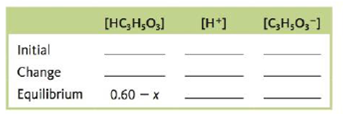# Consider a 0.60- M solution of HC 3 H 5 O 3 , lactic acid ( K a = 1.4 × 10 −4 ). a. Which of the following are major species in the solution? i. HC 3 H 5 O 3 ii. C 3 H 5 O 3 − iii. H + iv. H 2 O v. OH − b. Complete the following ICE table in terms of x, the amount (mol/L) of lactic acid that dissociates to reach equilibrium. c. What is the equilibrium concentration for C 3 H 3 O 3 − ? d. Calculate the pH of the solution.### Chemistry: An Atoms First Approach

2nd Edition
Steven S. Zumdahl + 1 other
Publisher: Cengage Learning
ISBN: 9781305079243

#### Solutions

Chapter
Section### Chemistry: An Atoms First Approach

2nd Edition
Steven S. Zumdahl + 1 other
Publisher: Cengage Learning
ISBN: 9781305079243
Chapter 13, Problem 164CWP
Textbook Problem
20 views

## Consider a 0.60-M solution of HC3H5O3, lactic acid (Ka = 1.4 × 10−4).a. Which of the following are major species in the solution?i. HC3H5O3ii. C3H5O3−iii. H+iv. H2Ov. OH−b. Complete the following ICE table in terms of x, the amount (mol/L) of lactic acid that dissociates to reach equilibrium.c. What is the equilibrium concentration for C3H3O3−?d. Calculate the pH of the solution.

(a)

Interpretation Introduction

Interpretation: The major species in the given solution of Lactic acid (CH3CH(OH)COOH) , missing values in the stated ICE table, the equilibrium concentration of C3H5O3 and the pH of solution is to be stated.

Concept introduction: Lactic acid is a α- hydroxyl acid and is more acidic than acetic acid due to the intramolecular hydrogen bonding. It is a chiral compound and dissociates in water as a weak acid.

To determine: The major species in the given solution of Lactic acid (CH3CH(OH)COOH) .

### Explanation of Solution

Explanation

The major species in solution are lactate ion (C3H5O3) and H+ .

The dissociation reaction of Lactic acid in aqueous solution is,

CH3

(b)

Interpretation Introduction

Interpretation: The major species in the given solution of Lactic acid (CH3CH(OH)COOH) , missing values in the stated ICE table, the equilibrium concentration of C3H5O3 and the pH of solution is to be stated.

Concept introduction: Lactic acid is a α- hydroxyl acid and is more acidic than acetic acid due to the intramolecular hydrogen bonding. It is a chiral compound and dissociates in water as a weak acid.

To determine: The missing values in the given ICE table.

(c)

Interpretation Introduction

Interpretation: The major species in the given solution of Lactic acid (CH3CH(OH)COOH) , missing values in the stated ICE table, the equilibrium concentration of C3H5O3 and the pH of solution is to be stated.

Concept introduction: Lactic acid is a α- hydroxyl acid and is more acidic than acetic acid due to the intramolecular hydrogen bonding. It is a chiral compound and dissociates in water as a weak acid.

To determine: The equilibrium concentration of C3H5O3 .

(d)

Interpretation Introduction

Interpretation: The major species in the given solution of Lactic acid (CH3CH(OH)COOH) , missing values in the stated ICE table, the equilibrium concentration of C3H5O3 and the pH of solution is to be stated.

Concept introduction: Lactic acid is a α- hydroxyl acid and is more acidic than acetic acid due to the intramolecular hydrogen bonding. It is a chiral compound and dissociates in water as a weak acid.

### Still sussing out bartleby?

Check out a sample textbook solution.

See a sample solution

#### The Solution to Your Study Problems

Bartleby provides explanations to thousands of textbook problems written by our experts, many with advanced degrees!

Get Started

Find more solutions based on key concepts
What behaviors would be most helpful in preventing osteoporosis?

Understanding Nutrition (MindTap Course List)

A benefit to health is seen when is used in place of in the diet. a. saturated fat/mono unsaturated fat b. satu...

Nutrition: Concepts and Controversies - Standalone book (MindTap Course List)

Chemistry In Focus

What are sister chromatids?

Human Heredity: Principles and Issues (MindTap Course List)

True or false? Some protists start out life with no nucleus.

Biology: The Unity and Diversity of Life (MindTap Course List)

What are the Milky Way Galaxys spiral arms?

Foundations of Astronomy (MindTap Course List)

Who was the next captain to circumnavigate Earth?

Oceanography: An Invitation To Marine Science, Loose-leaf Versin

A block of unknown mass is attached to a spring with a spring constant of 6.50 N/m and undergoes simple harmoni...

Physics for Scientists and Engineers, Technology Update (No access codes included)